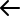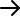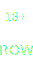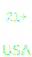Tips On How To Read Odds To Help You Make Winning Bets

## Explore our knowledge baseWhen starting to wager money on sports, you will see some interesting numbers in front of a team or player. They might even be accompanied by a plus or minus sign.

What do these numbers and signs mean? That is a very good question that a lot of newer sports bettors need answering. Understanding how to read these odds will make for successful betting.

## Top Tips For Reading Odds

You do not have to be super successful at math to understand betting odds. But you do need to understand the odds of probabilities and what they are implying to make the most of your bets.

Some common types of bets you will come across in sports betting are future bets, point spread bet and moneyline bets among others.

The top tips for how to read odds are:

• Basics of the three main odds
• Calculating implied probability
• Value bets

## Basics of the Three Main Odds

American Odds

The most common odds you will come across are going to be the American odds. Let us look at the following example to show how they work:

• Golden State Warriors: +120
• Los Angeles Lakers: -150

As you can see, the Warriors have a plus sign and the number 120. They are considered the underdog, or least likely to win. This means that for every \$100 placed on them, and if they win, you will receive \$120.

For the Lakers, they have the minus sign and the number 150. They are considered the favorite, or the most likely to win. This means that for every \$150 placed on them, and if they win, you will receive \$100.

Decimal Odds

These odds require you do just a little bit of math to convert. Do not be scared away at the math part, because this is easy to figure. Let us say that Team A has odds of 2.5.

For every \$1 you bet on Team A, you would get \$2.50. So, a \$100 bet would be \$250 total, or \$150 profit, and your \$100 original stake back.

Fractional Odds

If you come across any European odds or sporting events, you will likely see fractional odds. These odds are exactly as they sound, with fractions.

Let us say that Team A has odds of 3/2 to win a race.

With fractional odds, your top or first number given is the profit, and the bottom or second number is the proportion to the stake. This means that for every \$2 you bet, you would get a return of \$3 in profit.

So, a \$100 bet for this scenario would be a return of \$150.

## Calculating Implied Probability

As mentioned above, you do not have to be excellent at math to know how to read odds. However, by calculating the implied probability of an event, you can see how likely the event will turn out in a win or a loss.

The positive equation looks like this :

• Implied probability = 100 divided by (positive odds + 100)

So using the example above for the Warriors, they had a +120.

• Implied probability = 100 divided by (120 + 100)
• Implied probability = 100 divided by 220
• Implied probability = 0.45

This means that the likelihood of the Warriors winning the game is roughly at a 45 percent chance.

The negative equation looks like this :

• Implied probability = negative odds divided by (negative odds – 100)

Again using the example from above, the Lakers had -150.

• Implied probability = -150 divided by (-150 – 100)
• Implied probability = -150 divided by -250
• Implied probability = 0.6

This means that the likelihood of the Lakers winning the game is roughly at a 60 percent chance.

By using these equations to your advantage, you can see the probabilities in real-time and get a good estimate of how much money you are betting on the underdog or the favorite.

## Value Bets

Finding a value in a bet is very useful in long term betting success. Since we have now read the odds and calculated the implied probability, we can see if there is value in the teams.

If you think that the Warriors are better than their 45 percent probability to win, you should bet on them. Unless you believe that the Lakers are still better at their 60 percent chance to win, you should bet on them.

## How To Read Odds FAQ

What if I bet against the odds?
+

Let us say that you are betting on the Blackhawks to win the Stanley Cup, and they are the underdog at +300. If you bet \$100, you would gain an additional \$300 if they win.

To put this in another light, the odds are that you are betting that they are three times more likely to lose than win. This is why the payouts are nicer.

Can I get help calculating the odds?
+

If you are still having trouble calculating the implied probability or anything else, you can look up a free odds calculator and plug those numbers into it. Your sportsbook might also have one built into their website, so check around there too.

Why do the odds change so much?
+

Odds for sporting events are always subject to changes. Since it has a lot of people betting on the event, the lines will move slightly up and down a lot until the event begins. The market is trying to keep both sides fair when it comes to wagering money down.Get all the latest sports news, expert tips and reviews.